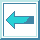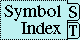### 5.1.2.2 Function Call Forms as Places

A function form can be used as a place if it falls into one of the following categories:

* A function call form whose first element is the name of any one of the functions in the next figure.

```aref    cdadr                    get
bit     cdar                     gethash
caaaar  cddaar                   logical-pathname-translations
caaar   cddar                    ninth
caar    cddr                     row-major-aref
car     fdefinition              svref
cdaaar  fifth                    symbol-function
cdaar   find-class               symbol-value
```

Figure 5-7. Functions that setf can be used with---1

In the case of subseq, the replacement value must be a sequence whose elements might be contained by the sequence argument to subseq, but does not have to be a sequence of the same type as the sequence of which the subsequence is specified. If the length of the replacement value does not equal the length of the subsequence to be replaced, then the shorter length determines the number of elements to be stored, as for replace.

* A function call form whose first element is the name of a selector function constructed by defstruct. The function name must refer to the global function definition, rather than a locally defined function.

* A function call form whose first element is the name of any one of the functions in the next figure, provided that the supplied argument to that function is in turn a place form; in this case the new place has stored back into it the result of applying the supplied ``update'' function.

```Function name  Argument that is a place  Update function used
ldb            second                    dpb
getf           first                     implementation-dependent
```

Figure 5-8. Functions that setf can be used with---2 During the setf expansion of these forms, it is necessary to call get-setf-expansion in order to figure out how the inner, nested generalized variable must be treated.

The information from get-setf-expansion is used as follows.

ldb

In a form such as:

(setf (ldb byte-spec place-form) value-form)

the place referred to by the place-form must always be both read and written; note that the update is to the generalized variable specified by place-form, not to any object of type integer.

Thus this setf should generate code to do the following:

1. Evaluate byte-spec (and bind it into a temporary variable).
2. Bind the temporary variables for place-form.
3. Evaluate value-form (and bind its value or values into the store variable).
4. Do the read from place-form.
5. Do the write into place-form with the given bits of the integer fetched in step 4 replaced with the value from step 3.

If the evaluation of value-form in step 3 alters what is found in place-form, such as setting different bits of integer, then the change of the bits denoted by byte-spec is to that altered integer, because step 4 is done after the value-form evaluation. Nevertheless, the evaluations required for binding the temporary variables are done in steps 1 and 2, and thus the expected left-to-right evaluation order is seen. For example:

``` (setq integer #x69) =>  #x69
(rotatef (ldb (byte 4 4) integer)
(ldb (byte 4 0) integer))
integer =>  #x96
;;; This example is trying to swap two independent bit fields
;;; in an integer.  Note that the generalized variable of
;;; interest here is just the (possibly local) program variable
;;; integer.
```

This case is the same as ldb in all essential aspects.

getf

In a form such as:

(setf (getf place-form ind-form) value-form)

the place referred to by place-form must always be both read and written; note that the update is to the generalized variable specified by place-form, not necessarily to the particular list that is the property list in question.

Thus this setf should generate code to do the following:

1. Bind the temporary variables for place-form.
2. Evaluate ind-form (and bind it into a temporary variable).
3. Evaluate value-form (and bind its value or values into the store variable).
4. Do the read from place-form.
5. Do the write into place-form with a possibly-new property list obtained by combining the values from steps 2, 3, and 4. (Note that the phrase ``possibly-new property list'' can mean that the former property list is somehow destructively re-used, or it can mean partial or full copying of it. Since either copying or destructive re-use can occur, the treatment of the resultant value for the possibly-new property list must proceed as if it were a different copy needing to be stored back into the generalized variable.)

If the evaluation of value-form in step 3 alters what is found in place-form, such as setting a different named property in the list, then the change of the property denoted by ind-form is to that altered list, because step 4 is done after the value-form evaluation. Nevertheless, the evaluations required for binding the temporary variables are done in steps 1 and 2, and thus the expected left-to-right evaluation order is seen.

For example:

``` (setq s (setq r (list (list 'a 1 'b 2 'c 3)))) =>  ((a 1 b 2 c 3))
(setf (getf (car r) 'b)
(progn (setq r nil) 6)) =>  6
r =>  NIL
s =>  ((A 1 B 6 C 3))
;;; Note that the (setq r nil) does not affect the actions of
;;; the SETF because the value of R had already been saved in
;;; a temporary variable as part of the step 1. Only the CAR
;;; of this value will be retrieved, and subsequently modified
;;; after the value computation.
```

The following X3J13 cleanup issues, not part of the specification, apply to this section: